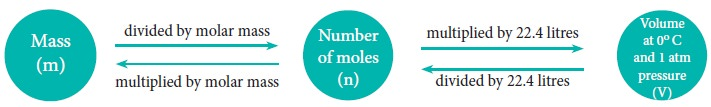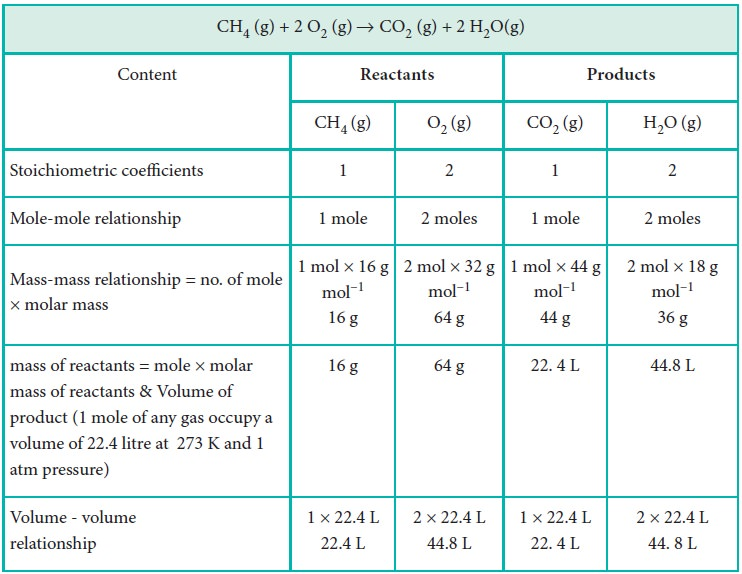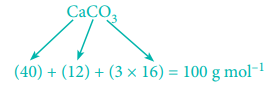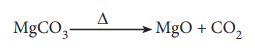Home | | Chemistry 11th std | Stoichiometric Calculations

# Stoichiometric Calculations

Stoichiometry is the quantitative relationship between reactants and products in a balanced chemical equation in moles.

Stoichiometric Calculations:

Stoichiometry is the quantitative relationship between reactants and products in a balanced chemical equation in moles. The quantity of reactants and products can be expressed in moles or in terms of mass unit or as volume. These three units are inter convertible.Let us explain these conversions by considering the combustion reaction of methane as an example. The balanced chemical equation is,

CH4 (g) + 2 O2 (g)  → CO2 (g) + 2 H2O(g)Calculations based on Stoichiometry

1. How many moles of hydrogen is required to produce 10 moles of ammonia ?

The balanced stoichiometric equation for the formation of ammonia is

N2(g) + 3 H2 (g)  → 2 NH3 (g)

As per the stoichiometric equation, to produce 2 moles of ammonia, 3 moles of hydrogen are required

∴ to produce 10 moles of ammonia,= 15 moles of hydrogen are required.

2. Calculate the amount of water produced by the combustion of 32 g of methane.

CH4(g) + 2O2(g) → CO2(g) + 2H2O(g)

As per the stoichiometric equation,

Combustion of 1 mole (16 g) CH4 produces 2 moles (2 × 18 = 36 g) of water.Combustion of 32 g CH4 produces3. How much volume of carbon dioxide is produced when 50 g of calcium carbonate is heated completely under standard conditions?

The balanced chemical equation is,As per the stoichiometric equation,

1 mole (100g) CaCO3 on heating produces 1 mole CO2At STP, 1 mole of CO2 occupies a volume of 22.7 litres

At STP, 50 g of CaCO3 on heating produces,4. How much volume of chlorine is required to form 11.2 L of HCl at 273 K and 1 atm pressure ?

The balanced equation for the formation of HCl is

H2 (g) + Cl2 (g) → 2 HCl (g)

As per the stoichiometric equation, under given conditions,

To produce 2 moles of HCl, 1 mole of chlorine gas is required

To produce 44.8 litres of HCl, 22.4 litres of chlorine gas are required.

To produce 11.2 litres of HCl,= 5.6 litres of chlorine are required.

5. Calculate the percentage composition of the elements present in magnesium carbonate. How many kilogram of CO2 can be obtained by heating 1 kg of 90 % pure magnesium carbonate.

The balanced chemical equation isMolar mass of MgCO3 is 84 g mol–1.

84 g MgCO3 contain 24 g of Magnesium.

100 g of MgCO3 contain= 28.57 g Mg.

i.e. percentage of magnesium

= 28.57 %.

84 g MgCO3 contain 12 g of carbon∴ Percentage of carbon

=    14.29 %.

84 g MgCO3 contain 48 g of oxygen

∴ 100 g MgCO3 contains= 57.14 g of oxygen.

Percentage of oxygen

= 57.14 %.

As per the stoichiometric equation,

84 g of 100 % pure MgCO3 on heating gives 44 g of CO2.

1000 g of 90 % pure MgCO3 gives= 471.43 g of CO2

= 0.471 kg of CO2

Study Material, Lecturing Notes, Assignment, Reference, Wiki description explanation, brief detail
11th Chemistry : UNIT 1 : Basic Concepts of Chemistry and Chemical Calculations : Stoichiometric Calculations |